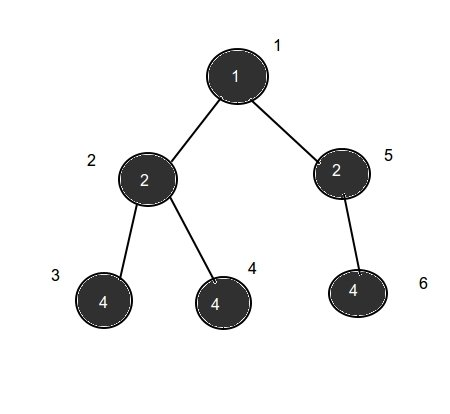Monk and Xor Tree
Tag(s):

Algorithms, Depth-first search, Easy, Graph, Graph Theory, Tree

Problem
Editorial
Analytics

Monk has become a master in graph and trees, so he is ready with a task for you.

Given a Tree T consisting of N nodes rooted at node 1, each node has a value, where the value of the $i^{th}$ node is $A[i]$.

In addition to the tree, you have also been given an integer K. Now, you need to find in this tree, the number of distinct pairs $(i,j)$ where node i is an ancestor of node j, and the XOR of the values of all nodes lying on the path from i to j is equal to K.

In short, you need to find the number of pairs $(i,j)$, where node i is an ancestor of j,
and :$\forall x$ lying on the path from i to j inclusive, $\oplus A[x]$ = K.

Here, the $\oplus$ symbol denotes the logical XOR operation.

Note:
A node x is considered an ancestor of another node y if x is parent of y or x is an ancestor of parent of y. For the purposes of this problem, we consider every node to be an ancestor of itself.

Can you do it ?

Input Format :

The first line contains 2 space separated integers N and K. The next line contains N space separated integers. where the $i^{th}$ integer denotes $A[i]$.

The next line contains $N-1$ space separated integers, where the $i^{th}$ integer denotes the parent of node $i+1$.

Output Format :

Print the required answer on a single line.

Constraints :

$1 \le N \le 10^5$

$0 \le K, A[i] \le 2^{20}$

$1 \le parent[i] < i$, where $2 \le i \le N$

SAMPLE INPUT
6 7
1 2 4 4 2 4
1 2 2 1 5
SAMPLE OUTPUT
3
ExplanationHere, the numbers written on the nodes represent their value, and the numbers written beside each node represents their index.

The pairs forming part of the answer are :

1. $(1,3)$ = $1 \oplus 2 \oplus 4=7$

2. $(1,4)$ = $1 \oplus 2 \oplus 4=7$

3. $(1,6)$ = $1 \oplus 2 \oplus 4=7$

Time Limit: 1.0 sec(s) for each input file.
Memory Limit: 256 MB
Source Limit: 1024 KB
Marking Scheme: Marks are awarded when all the testcases pass.
Allowed Languages: Bash, C, C++, C++14, Clojure, C#, D, Erlang, F#, Go, Groovy, Haskell, Java, Java 8, JavaScript(Rhino), JavaScript(Node.js), Julia, Kotlin, Lisp, Lisp (SBCL), Lua, Objective-C, OCaml, Octave, Pascal, Perl, PHP, Python, Python 3, R(RScript), Racket, Ruby, Rust, Scala, Swift, Swift-4.1, TypeScript, Visual Basic

CODE EDITOR

Initializing Code Editor...

ContributorChallenge Name

CodeMonk (Graph Theory Part I)

OTHER PROBLEMS OF THIS CHALLENGE
• Algorithms > Graphs
• Algorithms > Graphs
• Algorithms > Graphs
• Algorithms > Graphs